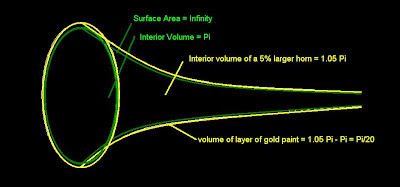## Saturday, February 13, 2010

### You Do The Math - Gabriel's Horn

Supposedly Gabriel's Horn is a paradox, where it's surface area is infinite, but it's interior volume is finite, specifically, Pi.

The horn is created when we take the graph of the equation Y=1/X, where X >= 1, and spin it about the X axis. This horn is infinitely long and skinny, and has a circular open end that is 2 units in diameter.

The paradox states that it takes Pi units of paint to fill the horn, yet it takes an infinite amount of paint to cover it's surface. How can that be?

It can't. The paradox assumes you lay down a 2 dimensional layer of paint. This is impossible! Mathematicians overlook the fact that a layer of paint is 3 dimensional.Therefore, the layer of paint has volume, specifically, the volume of a slightly larger horn minus the volume of the smaller, original horn.

Let's say the larger horn has 5% more volume. If so, then the volume of paint on the smaller horn is equal to 1.05Pi - Pi, which equals Pi/20

Therefore, it takes 20 times more paint to fill the horn than to cover it's outer surface.

No more paradox. Whew! For a minute there I was worried the Universe would explode.